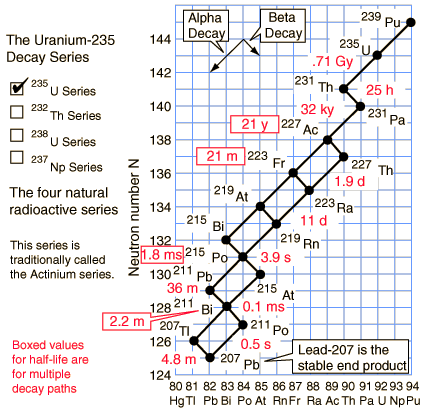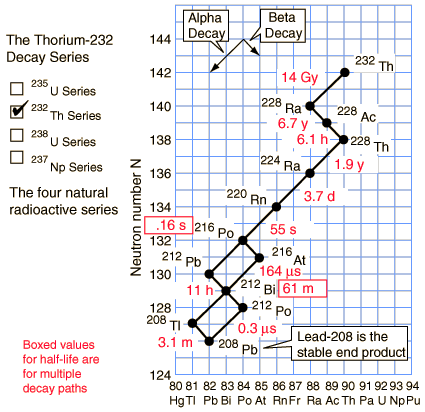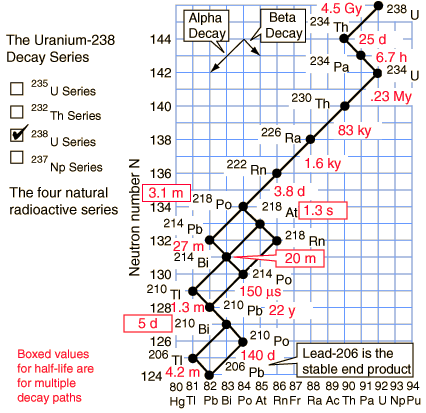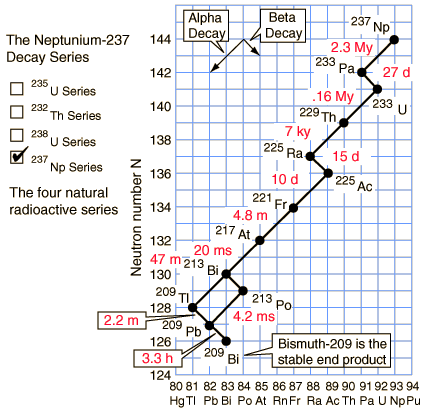1μs = 10-6s, 1 ms = 10-3s,1 My = 106y, 1 Gy = 109y
 Radioactivity Alpha decay Beta decay.
Index

 HyperPhysics***** Nuclear R Nave
Go Back1μs = 10-6s, 1 ms = 10-3s,1 My = 106y, 1 Gy = 109y
 Radioactivity Alpha decay Beta decay.
Index

 HyperPhysics***** Nuclear R Nave
Go Back1μs = 10-6s, 1 ms = 10-3s,1 My = 106y, 1 Gy = 109y
 Radioactivity Alpha decay Beta decay.
Index

 HyperPhysics***** Nuclear R Nave
Go Back1μs = 10-6s, 1 ms = 10-3s,1 My = 106y, 1 Gy = 109y

The members of this series are not presently found in nature because the half-life of the longest lived isotope in the series is short compared to the age of the earth.

 Radioactivity Alpha decay Beta decay.
Index

 HyperPhysics***** Nuclear R Nave
Go Back